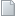Journal of Statistics Applications & Probability Letters An International JournalForthcomingComparison of redundant components allocation policies in series systems Abstract : Comparing different redundant components allocation policies in series systems is of great interest both in theory and in practice. This paper provides a simple criteria for comparing two policies in terms of reversed hazard rate ordering. We conjecture that this simple criteria is applicable in terms of likelihood ratio ordering, excepting some extreme situations. Computer simulations strongly support the conjecture.Estimating the parameters of an exponentiated inverted Weibull distribution under type-II censoring Abstract : The exponentiated inverted Weibull distribution is a generalization of the exponentiated inverted exponential distribution as well as the inverted Weibull distribution. In this paper, Bayes and classical estimators have been obtained for two parameters exponentiated inverted Weibull distribution when sample is avail- able from complete and type II censoring scheme. Several Bayesian estimates are obtained against di§erent symmetric and asymmetric loss functions such as squared error and LINEX. This was done with respect to the conjugate priors for two shape parameters. As expected, when the shape parameters are unknown, the closed-form expressions of the Bayes estimators cannot be obtained. We used an approximation based on the Lindley and Markov chain Monte Carlo method (MCMC) methods for obtaining Bayes estimates under these loss functions. All Bayesian estimates are compared with the corresponding maximum likelihood estimates numerically in terms of their mean square error values. Finally, real data sets are analyzed for the purpose of illustration.